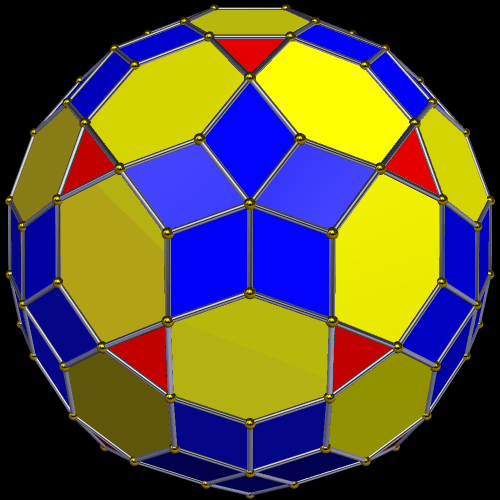Acronym ... Name icosa-expanded rhombic enneacontahedron© Vertex figure [r5], [r,h,3,h], [R2,H] General of army (is itself convex) Colonel of regiment (is itself locally convex)

The rhombs {(r,R)2} have vertex angles r = arccos(1/3) = 70.528779° resp. R = arccos(-1/3) = 109.471221°. Esp. rr : RR = sqrt(2) = 1.414214.
The hexagons {(h,h,H)2} have vertex angles h = arccos(-sqrt[(3-sqrt(5))/6]) = 110.905157° resp. H = arccos[-sqrt(5)/3] = 138.189685°.

This polyhedron is just the Stott expansion of the rhombic enneacontahedron wrt. its icosahedral vertices.

All a, b, c, and d edges, provided in the below description, only qualify as pseudo edges wrt. the full polyhedron.

Incidence matrix according to Dynkin symbol

abx3oco5ood&#zx   → height = 0,
a = 1+sqrt(20/3) = 3.581989,
b = HH = 1+(sqrt(5)-1)/sqrt(3) = 1.713644,
c = RR = 2/sqrt(3) = 1.154701,
d = hh = (1+sqrt(5))/sqrt(3) = 1.868345

o..3o..5o..     | 12  *  * |  5   0  0 |  5  0  0  [r5]
.o.3.o.5.o.     |  * 60  * |  1   2  0 |  2  1  0  [R2,H]
..o3..o5..o     |  *  * 60 |  0   2  2 |  1  2  1  [r,h,3,h]
----------------+----------+-----------+---------
oo.3oo.5oo.&#x  |  1  1  0 | 60   *  * |  2  0  0
.oo3.oo5.oo&#x  |  0  1  1 |  * 120  * |  1  1  0
..x ... ...     |  0  0  2 |  *   * 60 |  0  1  1
----------------+----------+-----------+---------
... oco ...&#xt |  1  2  1 |  2   2  0 | 60  *  *  {(r,R)2}
.bx ... .od&#zx |  0  2  4 |  0   4  2 |  * 30  *  {(h,h,H)2}
..x3..o ...     |  0  0  3 |  0   0  3 |  *  * 20